Here is code to perform 2D Frouier transforms on jepg files. fft.c is a C program to perform the Fast Fourier Transform. ft.c is a multi threaded 2D FFT considerably adapted from this.

The following shell commands build and demonstrate the code on the Mac (and some Linuxes). You will need h.h.

```javac cim.java
gcc fft.c -O3 -c -Wmost
gcc ft.c -O3 -Wmost -c -fnested-functions
java j2b th.jpg out.bma
java b2j out.bma out.jpg
gcc man.c fft.o ft.o -O3 -o trans -Wmost
./trans nop out.bma outt.bma 8
java b2j outt.bma outt.jpg
open outt.jpg```
The trans command reads and writes a file with a name ending in “.bma” in these demos. Such files hold raw 24 bit RGB pixel values preceded by image dimensions. In general the command:
`./trans op in.bma oqut.bma r`
transforms the input image to frequency space, calls the one of the functions, in the file man.c, and transforms back to image space and writes the output file. The parameter r is a floating point value used by most of the variations and is in units of pixels.
• op=“hop” merely replaces each Fourier term with its complex conjugate. This is the identity transformation on the image and shows that a great deal of the mathematical magic is working.
• op=“ct” eliminates all frequency components greater than 1/r.
• op=“Gauss” attenuates each frequency f by e—(f/r)2 yielding a Gaussian blur.
• op=“unf” multiplies each frequency component by the transform of a uniform circular disk of radius r. In effect this convolves the original image with that disk.
• op=“up” moves image up r pixels.

The high level mechanics of this process can be learned by examining which files are created by each command.

The file out.bma is produced to show that we can translate to and from the raw byte format with minimal damage. open out.jpg to see it.

To undo build and run files:
rm outt* trans *.o *.class out.bma

### Notes on the code in ft.c

The array ‘a’ is global and is accessed by most routines in files man.c and ft.c. It is conceived as a rectangular array of complex numbers with both dimensions rounded up to a power of two in order to accommodate the logic of the conventional fast Fourier transform, fft.c. In one execution of the main routine, it holds successively the red, green and blue color components and while holding them any transforms are applied to the array in place. Expressions such as a[n<<LX|m] would be written as a[n, m] in a language where 2D arrays were first class values.

The three 2D arrays cr, cg, cb are also byte per pixel arrays with as many elements and indexed the same as the complex array. They hold the segregated color components of the entire image. The gross information flow is: file to 3 byte arrays; to complex array a; to in-place transform of a; to one of the special routines in man.c; again to transform of a; to 3 byte arrays (scaled to peak value in a); to file. The code has been arranged to facilitate modifications such as averaging the input colors to produce a gray scale image, doing one transformation, and then using color to portray the vital convex numbers of the results of one transform.

Nested routine dc is executed once for each of the 3 primary colors. It moves the data from one of the one byte arrays into array a and does the transforms, and then back into the one byte arrays.

Routine pp(i, j) reports the value of a[i, j] for debugging and understanding. It has no side internal effects. d2Dft() causes the complex array to be transformed in place.

Routine fp(th, comment) scans the entire complex array and reports subscripts and values of components within th of the maximum magnitude. (“th” is a floating point real.) It returns the linear index of the max element.

The if(1) on the line with comment “Choice of two demos” selects the first piece of code which performs two 2D transforms calling routine manip between these two transforms. manip is supplied separately in another file such as mana.c which in this case replaces each complex number with its complex conjugate and that prevents the yield from being inverted. Many interesting experiments can be done by variations on routine manip.

If the 2nd demo is chosen (if(0)) the three colors are averaged for each input pixel and one transform is performed. Constant brightness color is used to code the complex number.

Other cruft is inherited from here.

#### Variations in man.c

There are several routines here, the nth of which us used with the shell command “./trans n … ”. The routine rm is the distance to the nearest interval end. In gcc if x is a complex expression then ~x is its complex conjugate. The complex conjugate of each frequency component is taken lest the logic of the FFT rotate the image by π.
 ./trans nop in out 0unchangedFT unmodified (except for conjugating each element to avoid rotation of image.) ./trans ct in out 8abrupt frequency cutoff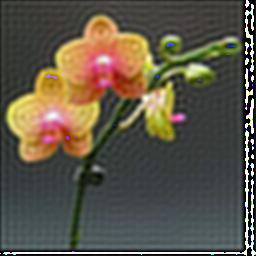All spatial frequency components greater than 1/r are removed. ./trans Gauss in out 8gaussian frequency attenuation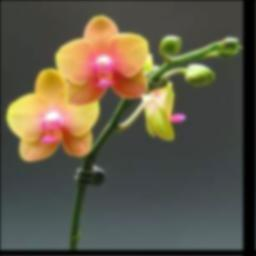attenuate each frequency f by e−(r*f)2. This is sometimes referred to as a Gaussian blur. ./trans unf in out 4Convolution with disk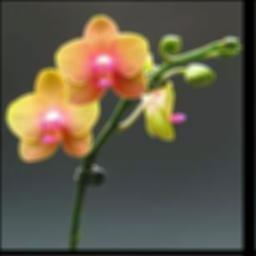Compute transform of uniform circular disk inorder to convolve via FFT. r = 4 here. ./trans up in out 100Vertical translation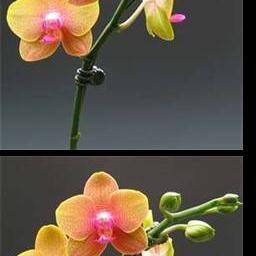Translates up r pixels with wraparound. ./trans db in out 100 47 0.30General translation with double exposure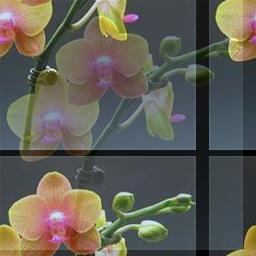2D translation with wraparound and double exposure with original position.
These images were produced by these commands:
```javac cim.java
gcc fft.c -O3 -c -Wmost
gcc ft.c -O3 -Wmost -c -fnested-functions
gcc man.c fft.o ft.o -O3 -o trans -Wmost
java j2b th.jpg out.bma
./trans nop out.bma outt.bma 0
java b2j outt.bma nop.jpg
./trans ct out.bma outt.bma 8
java b2j outt.bma ct.jpg
./trans Gauss out.bma outt.bma 8
java b2j outt.bma Gauss.jpg
./trans unf out.bma outt.bma 4
java b2j outt.bma unf.jpg
./trans up out.bma outt.bma 100
java b2j outt.bma up.jpg
./trans db out.bma outt.bma 100 47 0.30
java b2j outt.bma db.jpg
```

Digression: This reads a bma file, averages the three colors of each pixel, and writes a .jpg gray file.
```javac cim1.java
java b2j1 out.bma out1.jpg
open out1.jpg```

### Miscellaneous observations

Several browsers decide the format of an image file by looking in the file instead of either
• the extension in the file name, here “jpg”
• or the “Content-Type:” in the http header file.
The Java graphics package does also and you can provide a .png file in place of a .jpg file. It matters not what you call it. If you ask Safari to save an image file it volunteers the correct extension, regardless of the name by which it fetched the file.

### A bit of transform theory.

The four transforms above are each convolutions of the input file th.jpg and:
1. Dirac’s delta function, convolution with which is the identity.
2. The transform of a uniform circular disk of radius r. This transform is not always positive and the image may have negative pixels which show up as white in the margin.
3. The 2D circular Gaussian with standard deviation of r pixels. The Gaussian is perhaps the only positive function whose transform is also positive. This results in a positive convolution since we take all of the input pixels to be non-negative.
4. A uniform disk of radius r.
The ringing seen with the abrupt frequency cutoff is closely related to the Gibbs overshoot phenomenon. The gradual cutoff of the Gaussian blur eliminates the ringing just as Cesàro summation ameliorates Gibbs ringing.

### Transform Topology

The FFT is by nature periodic. As a consequence the 2D transform is on the 2D torus. Blurs bleed off the edge and return on the opposite edge. The black border added to round to a power of 2 may thus ameliorate unwanted effects. A color other than black could be chosen.
good general information
Fractal Noise; See nice animated .gif here.